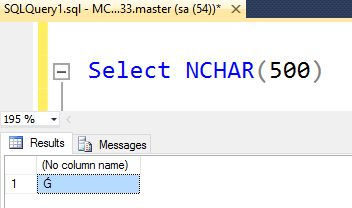In focus

# String Functions In SQL Server: Part One

## In this article you will learn about string functions in SQL Server. This is Part One of the series.

Avinash Thakur Apr 18, 2016

String functions (T-SQL) in SQL Server are as given below.
• ASCII
• CHAR
• CHARINDEX
• DIFFRENCE
• LEFT
• LEN
• LOWER
• LTRIM
• NCHAR
ASCII() : It is use to convert the number value of a specified character.

Example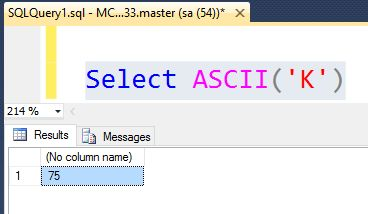CHARINDEX (char, varchar, start_index) : It is used to search specified characters into specified string from starting character index (start_index) to end of string length.

Example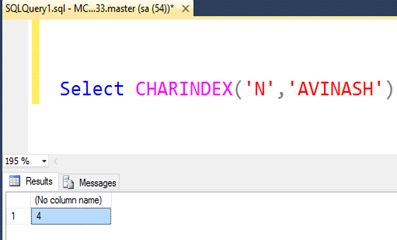DIFFRENCE
(varchar, varchar) : It returns integer values. In the real world this function is usable for search operations.

Return value types:
• 0 (Zero) represents no similarity between two strings.
• 4 represents strong similarity between two strings.
• 1, 2 and 3, represents very close but not strong similarity.
Example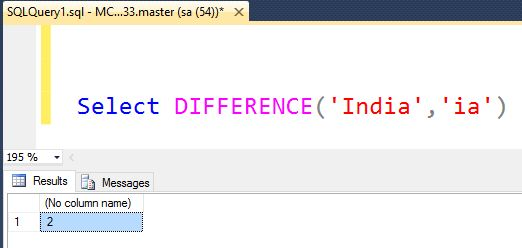LEFT (varchar, length) : It is used to return string from character index 0 to length value.

Example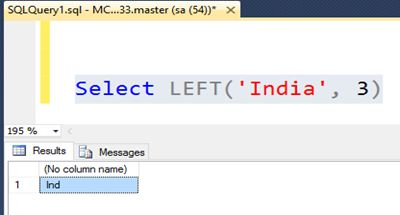LEN
(varchar) : It is used to return length of specified value as integer.

Example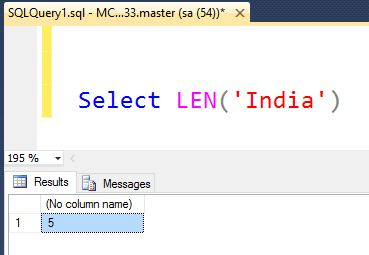LOWER
(varchar) : It is used to convert and returns lower case value of specified value.

ExampleLTRIM (varchar): It is used to remove spaces in specified value of the left.

Example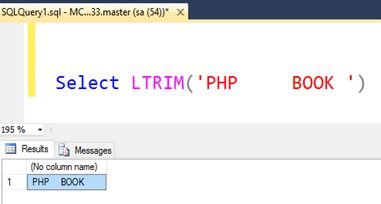CHAR
() : It is used to convert ASCII number to character value.

Example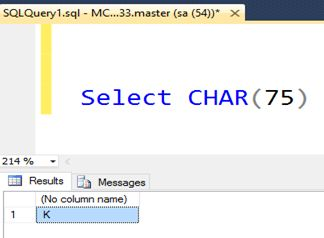NCHAR
(char_number) : It is the same as CHAR() function but this function uses UNICODE standard.

Example